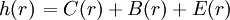# Difference between revisions of "Cluster diagrams"

## Chain clusters

A chain is a cluster with at least one node. All routes from one base point to the other pass through at least one nodal field point. Cutting at this point separates the diagram into two parts, each of which contains a base point. A simple chain is one in which every field point is a node. A netted chain is one that can be formed from a simple chain by adding not more than one field point across each link of the simple chain.

## Bundles

A bundle is a parallel collection of links. It has no nodes since there is always more than one independent path from one base point to the other.

## Elementary Clusters

An elementary cluster is that which is neither a chain or a bundle.$\left.h(r)\right. = C(r) + B(r) + E(r)$

where C(r) is the set of chain clusters, B(r) is the set of bundles, and E(r) is the set of elementary clusters (Eq. 5.2 Ref. 1). Similarly,$\left.c(r)\right. = B(r) + E(r)$